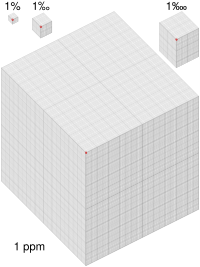# Basis point facts for kids

Kids Encyclopedia Facts
Quick facts for kids
per ten thousand sign
Punctuation
apostrophe   '
brackets [ ]  ( )  { }  ⟨ ⟩
colon :
comma ,  ،
dash ‒  –  —  ―
ellipsis   ...  . . .
exclamation mark  !
full stop, period .
guillemets ‹ ›  « »
hyphen
hyphen-minus -
question mark  ?
quotation marks ‘ ’  “ ”  ' '  " "
semicolon ;
slash, stroke, solidus /
Word dividers
interpunct ·
space
General typography
ampersand &
asterisk *
at sign @
backslash \
basis point
bullet
caret ^
dagger † ‡ ⹋
degree °
ditto mark ” 〃
equals sign =
inverted exclamation mark ¡
inverted question mark ¿
komejirushi, kome, reference mark
multiplication sign ×
number sign, pound, hash #
numero sign
obelus ÷
ordinal indicator º ª
percent, per mil  % ‰
pilcrow
plus, minus + −
plus-minus, minus-plus ± ∓
prime
section sign §
tilde ~
underscore, understrike _
vertical bar, pipe, broken bar |    ¦
Intellectual property
copyleft 🄯
service mark
Currency
currency sign ¤

؋ ​₳ ​ ฿ ​ ​ ₵ ​¢ ​₢ ​ \$ ​₫ ​₯ ​֏ ​ ₠ ​ ​ ƒ ​₣ ​ ₲ ​ ₴ ​ ₭ ​ ​₾ ​ ​ ₧ ​₱ ​₰ ​£ ​ 元 圆 圓 ​៛ ​₽ ​₹ ₨ ​ ₪ ​ ​₸ ​₮ ​ ₩ ​ ¥ ​

Uncommon typography
asterism
fleuron, hedera
index, fist
interrobang
irony punctuation
lozenge
tie
Related
In other scripts
• Chinese
• Hebrew
• Japanese
• Korean
• Category
• Book

A per ten thousand sign or basis point (often denoted as bp, often pronounced as "bip" or "beep") is (a difference of) one hundredth of a percent or equivalently one ten thousandth. The related concept of a permyriad is literally one part per ten thousand. Figures are commonly quoted in basis points in finance, especially in fixed income markets.

## DefinitionVisualisation of 1%, 1‰, 1‱ and 1 ppm as the fraction of the red cube to its respective block (larger version)
1 basis point = (a difference of) 1 permyriad or one-hundredth of one percent.
1 bp = (a difference of) 1‱ or 0.01% or 0.1‰ or 10−4 or 110,000 or 0.0001.
100 bp = (a difference of) 1% or 10‰ of 100‱.

Basis points are used as a convenient unit of measurement in contexts where percentage differences of less than 1% are discussed. The most common example is interest rates, where differences in interest rates of less than 1% per year are usually meaningful to talk about. For example, a difference of 0.10 percentage points is equivalent to a change of 10 basis points (e.g., a 4.67% rate increases by 10 basis points to 4.77%). In other words, an increase of 100 basis points means a rise by 1 percentage point.

Like percentage points, basis points avoid the ambiguity between relative and absolute discussions about interest rates by dealing only with the absolute change in numeric value of a rate. For example, if a report says there has been a "1% increase" from a 10% interest rate, this could refer to an increase either from 10% to 10.1% (relative, 1% of 10%), or from 10% to 11% (absolute, 1% plus 10%). However, if the report says there has been a "100 basis point increase" from a 10% interest rate, then the interest rate of 10% has increased by 1.00% (the absolute change) to an 11% rate.

It is common practice in the financial industry to use basis points to denote a rate change in a financial instrument, or the difference (spread) between two interest rates, including the yields of fixed-income securities.

Since certain loans and bonds may commonly be quoted in relation to some index or underlying security, they will often be quoted as a spread over (or under) the index. For example, a loan that bears interest of 0.50% per annum above the London Interbank Offered Rate (LIBOR) is said to be 50 basis points over LIBOR, which is commonly expressed as "L+50bps" or simply "L+50".

The term "basis point" has its origins in trading the "basis" or the spread between two interest rates. Since the basis is usually small, these are quoted multiplied up by 10,000, and hence a "full point" movement in the "basis" is a basis point. Contrast with pips in FX forward markets. En lieu of referencing individual basis points for larger percentages, the below terms have been gaining traction and use in the financial industry.

1 "MegaBip" = 10 bps = 00.1%

1 "UltraBip" = 100 bps = 01.0%

1 "GigaBip" = 1000 bps = 10.0%

Expense ratios of investment funds are often quoted in basis points.Basis point Facts for Kids. Kiddle Encyclopedia.